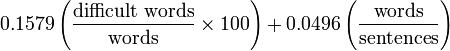# Readability Level by Dale-Chall Score

Dale-Chall score formula is used to assess the readability level of a given text, which is described below:Difficult words do not belong to the list of 3,000 familiar words. The formula adds 3.6365 to the raw score if the percentage of difficult words is greater than 5% to get the adjusted score. More information about the Dale-Chall readability score can be found here.
The mapping of Dale-Chall score to Readability level:
4.9 or lower easily understood by an average 4th-grade student or lower
5.0–5.9 easily understood by an average 5th or 6th-grade student
6.0–6.9 easily understood by an average 7th or 8th-grade student
7.0–7.9 easily understood by an average 9th or 10th-grade student
8.0–8.9 easily understood by an average 11th or 12th-grade student
9.0–9.9 easily understood by an average 13th to 15th-grade (college) student
10.0 or higher easily understood by an average college graduate

Below section also lists several other readability statistics this tool provides, including the formula and the score description. These formulas may deviate from other sources, please correct or suggest by contacting the author of Word Counter.

The formulas use some information about the text with abrreviates:

C
Number of characters
W
Number of words
UW
Number of unique words
DW
Number of difficult words
EW
Number of easy words
SW
Number of short words
LW
Number of long words
S
Number of sentences
SY
Number of syllables
M
Number of monosyllabic words (1 syllable)
PSY
Number of polysyllabic words (3 syllables or more)
Formulas
(4.71 x (C / W)) + (0.5 x (W / S)) - 21.43
Coleman-Liau Index
(5.89 x (C / W)) - (0.3 x (S / W)) - 15.8
Coleman-Liau Grade Level: = Coleman-Liau Index - 5